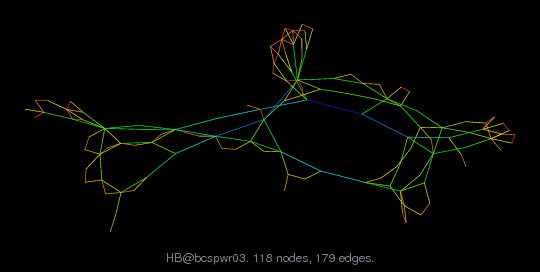Matrix: HB/bcspwr03

Description: SYMMETRIC STRUCTURE OF 118 BUS IEEE STANDARD TEST CASE POWER NETWORK(undirected graph drawing)• Matrix group: HB
• download as a MATLAB mat-file, file size: 1 KB. Use UFget(15) or UFget('HB/bcspwr03') in MATLAB.

 Matrix properties number of rows 118 number of columns 118 nonzeros 476 structural full rank? yes structural rank 118 # of blocks from dmperm 1 # strongly connected comp. 1 explicit zero entries 0 nonzero pattern symmetry symmetric numeric value symmetry symmetric type binary structure symmetric Cholesky candidate? yes positive definite? no

 author B. Dembart, J. Lewis editor I. Duff, R. Grimes, J. Lewis date 1981 kind power network problem 2D/3D problem? no

 Ordering statistics: result nnz(chol(P*(A+A'+s*I)*P')) with AMD 383 Cholesky flop count 1.3e+03 nnz(L+U), no partial pivoting, with AMD 648 nnz(V) for QR, upper bound nnz(L) for LU, with COLAMD 481 nnz(R) for QR, upper bound nnz(U) for LU, with COLAMD 927

 SVD-based statistics: norm(A) 5.1053 min(svd(A)) 0.0101817 cond(A) 501.42 rank(A) 118 sprank(A)-rank(A) 0 null space dimension 0 full numerical rank? yes

 singular values (MAT file): click here SVD method used: s = svd (full (A)) ; status: ok